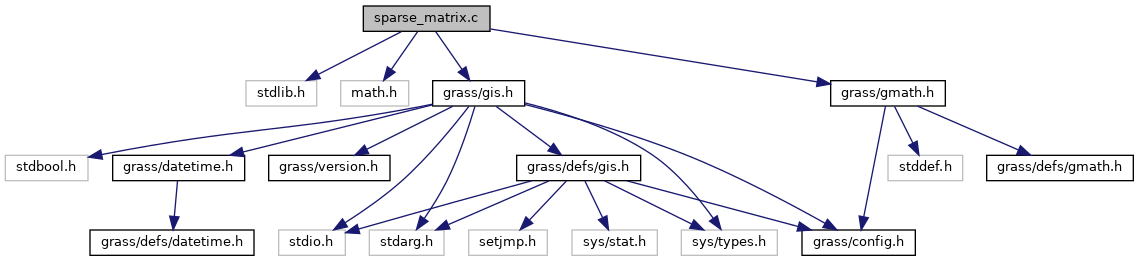GRASS GIS 7 Programmer's Manual  7.9.dev(2021)-e5379bbd7
sparse_matrix.c File Reference
`#include <stdlib.h>`
`#include <math.h>`
`#include <grass/gmath.h>`
`#include <grass/gis.h>`
Include dependency graph for sparse_matrix.c:Go to the source code of this file.

## Functions

int G_math_add_spvector (G_math_spvector **Asp, G_math_spvector *spvector, int row)
Adds a sparse vector to a sparse matrix at position row. More...

G_math_spvector ** G_math_alloc_spmatrix (int rows)
Allocate memory for a sparse matrix. More...

G_math_spvectorG_math_alloc_spvector (int cols)
Allocate memory for a sparse vector. More...

void G_math_free_spvector (G_math_spvector *spvector)
Release the memory of the sparse vector. More...

void G_math_free_spmatrix (G_math_spvector **Asp, int rows)
Release the memory of the sparse matrix. More...

void G_math_print_spmatrix (G_math_spvector **Asp, int rows)
print the sparse matrix Asp to stdout More...

double ** G_math_Asp_to_A (G_math_spvector **Asp, int rows)
Convert a sparse matrix into a quadratic matrix. More...

double ** G_math_Asp_to_sband_matrix (G_math_spvector **Asp, int rows, int bandwidth)
Convert a symmetric sparse matrix into a symmetric band matrix. More...

G_math_spvector ** G_math_A_to_Asp (double **A, int rows, double epsilon)
Convert a quadratic matrix into a sparse matrix. More...

G_math_spvector ** G_math_sband_matrix_to_Asp (double **A, int rows, int bandwidth, double epsilon)
Convert a symmetric band matrix into a sparse matrix. More...

void G_math_Ax_sparse (G_math_spvector **Asp, double *x, double *y, int rows)
Compute the matrix - vector product of sparse matrix **Asp and vector x. More...

## ◆ G_math_A_to_Asp()

 G_math_spvector** G_math_A_to_Asp ( double ** A, int rows, double epsilon )

Convert a quadratic matrix into a sparse matrix.

This function is multi-threaded with OpenMP. It creates its own parallel OpenMP region.

Parameters
 A (double **) rows (int) epsilon (double) – non-zero values are greater then epsilon
Returns
(G_math_spvector **)

Definition at line 253 of file sparse_matrix.c.

 int G_math_add_spvector ( G_math_spvector ** Asp, G_math_spvector * spvector, int row )

Adds a sparse vector to a sparse matrix at position row.

Return 1 for success and -1 for failure

Parameters
 Asp G_math_spvector ** spvector G_math_spvector * row int
Returns
int 1 success, -1 failure

Definition at line 35 of file sparse_matrix.c.

References G_debug(), and NULL.

## ◆ G_math_alloc_spmatrix()

 G_math_spvector** G_math_alloc_spmatrix ( int rows )

Allocate memory for a sparse matrix.

Parameters
 rows int
Returns
G_math_spvector **

Definition at line 58 of file sparse_matrix.c.

References G_calloc, and G_debug().

## ◆ G_math_alloc_spvector()

 G_math_spvector* G_math_alloc_spvector ( int cols )

Allocate memory for a sparse vector.

Parameters
 cols int
Returns
G_math_spvector *

Definition at line 76 of file sparse_matrix.c.

Referenced by N_assemble_les_2d_param(), and N_assemble_les_3d_param().

## ◆ G_math_Asp_to_A()

 double** G_math_Asp_to_A ( G_math_spvector ** Asp, int rows )

Convert a sparse matrix into a quadratic matrix.

This function is multi-threaded with OpenMP. It creates its own parallel OpenMP region.

Parameters
 Asp (G_math_spvector **) rows (int)
Returns
(double **)

Definition at line 179 of file sparse_matrix.c.

## ◆ G_math_Asp_to_sband_matrix()

 double** G_math_Asp_to_sband_matrix ( G_math_spvector ** Asp, int rows, int bandwidth )

Convert a symmetric sparse matrix into a symmetric band matrix.

```Symmetric matrix with bandwidth of 3

5 2 1 0
2 5 2 1
1 2 5 2
0 1 2 5

will be converted into the band matrix

5 2 1
5 2 1
5 2 0
5 0 0```
Parameters
 Asp (G_math_spvector **) rows (int) bandwidth (int)
Returns
(double **) the resulting ymmetric band matrix [rows][bandwidth]

Definition at line 221 of file sparse_matrix.c.

## ◆ G_math_Ax_sparse()

 void G_math_Ax_sparse ( G_math_spvector ** Asp, double * x, double * y, int rows )

Compute the matrix - vector product of sparse matrix **Asp and vector x.

This function is multi-threaded with OpenMP and can be called within a parallel OpenMP region.

y = A * x

Parameters
 Asp (G_math_spvector **) x (double) *) y (double * ) rows (int)
Returns
(void)

Definition at line 363 of file sparse_matrix.c.

Referenced by N_les_integrate_dirichlet_2d(), and N_les_integrate_dirichlet_3d().

## ◆ G_math_free_spmatrix()

 void G_math_free_spmatrix ( G_math_spvector ** Asp, int rows )

Release the memory of the sparse matrix.

Parameters
 Asp G_math_spvector ** rows int
Returns
void

Definition at line 121 of file sparse_matrix.c.

References G_free(), G_math_free_spvector(), and NULL.

Referenced by N_free_les().

## ◆ G_math_free_spvector()

 void G_math_free_spvector ( G_math_spvector * spvector )

Release the memory of the sparse vector.

Parameters
 spvector G_math_spvector *
Returns
void

Definition at line 98 of file sparse_matrix.c.

References G_free(), G_math_spvector::index, NULL, and G_math_spvector::values.

Referenced by G_math_free_spmatrix().

## ◆ G_math_print_spmatrix()

 void G_math_print_spmatrix ( G_math_spvector ** Asp, int rows )

print the sparse matrix Asp to stdout

Parameters
 Asp (G_math_spvector **) rows (int)
Returns
void

Definition at line 146 of file sparse_matrix.c.

References G_math_spvector::cols.

## ◆ G_math_sband_matrix_to_Asp()

 G_math_spvector** G_math_sband_matrix_to_Asp ( double ** A, int rows, int bandwidth, double epsilon )

Convert a symmetric band matrix into a sparse matrix.

WARNING: This function is experimental, do not use. Only the upper triangle matrix of the band strcuture is copied.

Parameters
 A (double **) the symmetric band matrix rows (int) bandwidth (int) epsilon (double) – non-zero values are greater then epsilon
Returns
(G_math_spvector **)

Definition at line 304 of file sparse_matrix.c.